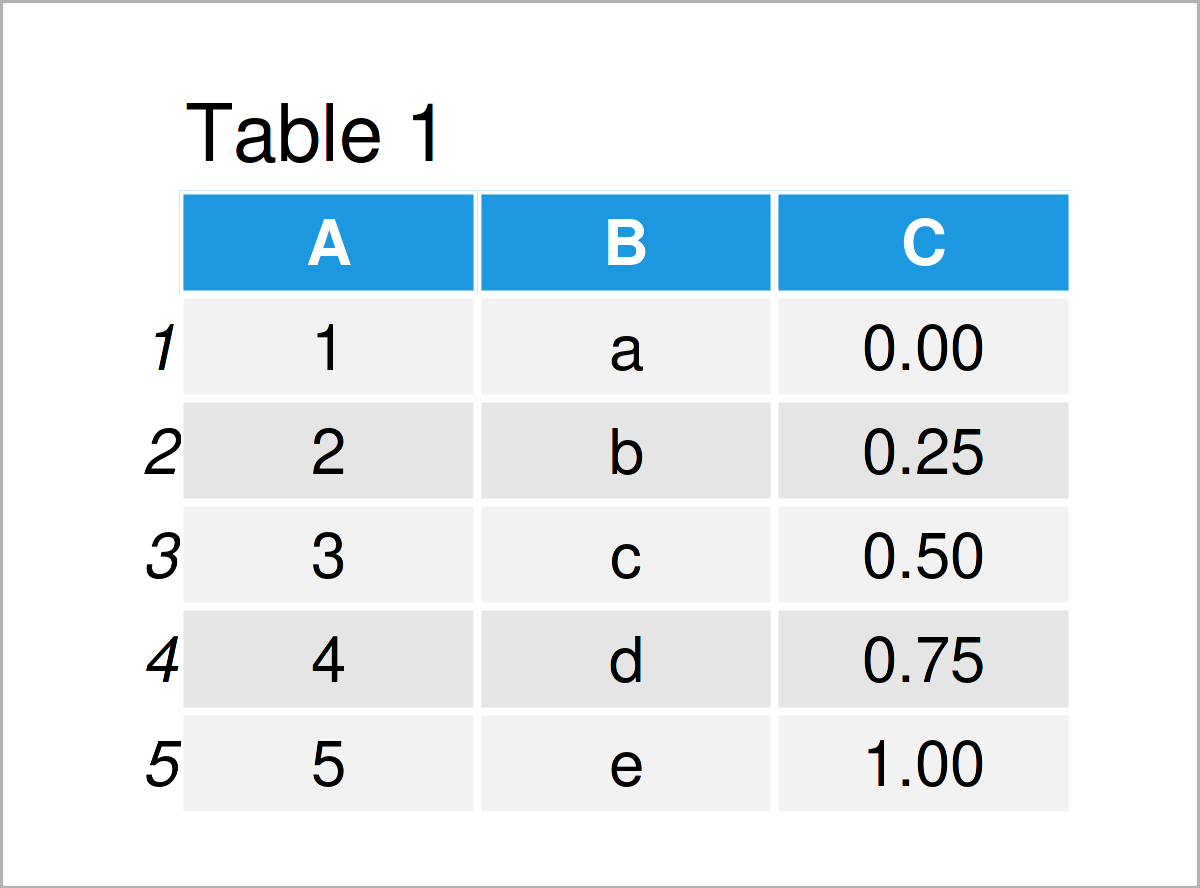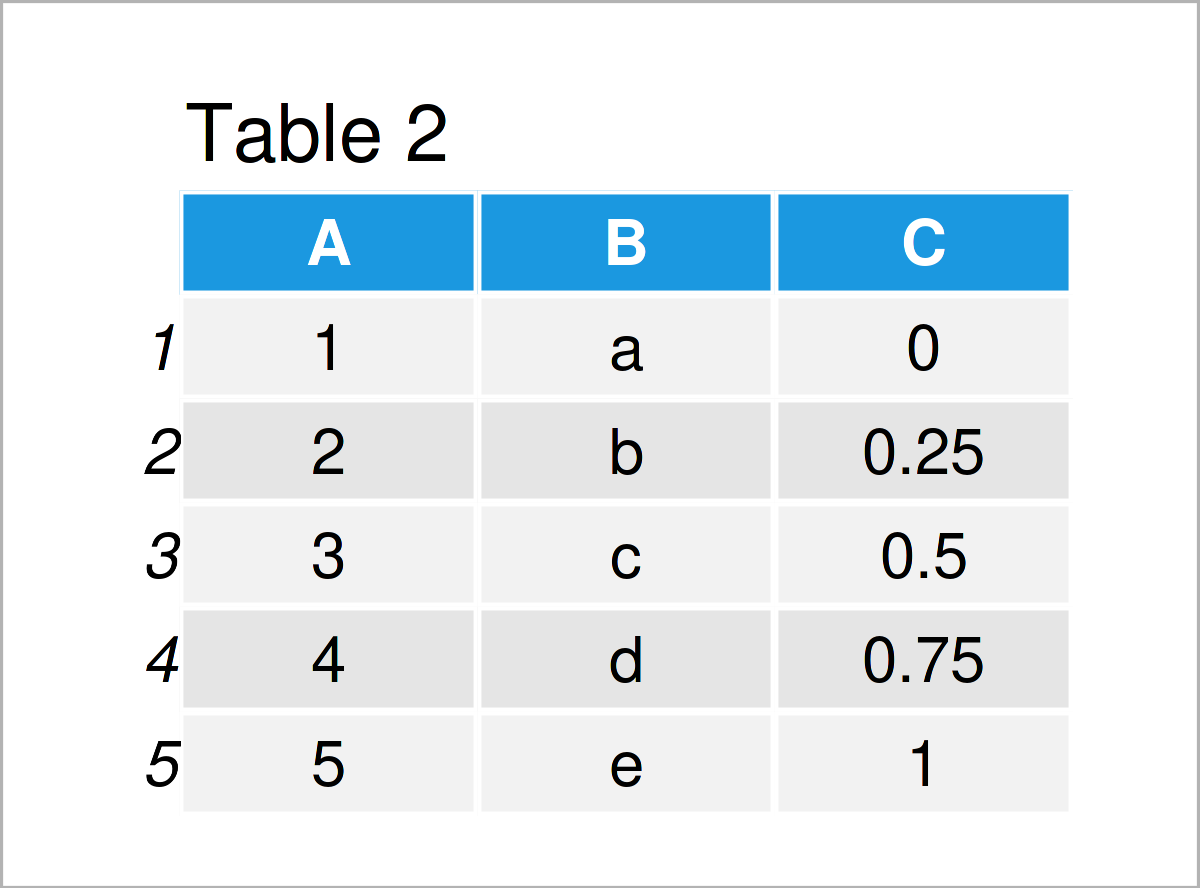# Convert List to data.table in R (2 Examples)

In this post, you’ll learn how to transform a list into a data.table in R programming. Make sure to also visit our data.table page here, where we give an overview of the package and many related tutorials.

This tutorial consists of the following content blocks:

It’s time to dive into the examples.

## Example Data & Packages

First, we need to install and load the data.table software package:

```install.packages("data.table")             # Install & load data.table package
library("data.table")```

Next, we construct some example data:

```l_1 <- list( A = 1:5,
B = letters[1:5],
C = seq(0, 1, length.out = 5) )
l_1
# \$A
#  1 2 3 4 5
#
# \$B
#  "a" "b" "c" "d" "e"
#
# \$C
#  0.00 0.25 0.50 0.75 1.00```

Have a look at the previous RStudio console output. It shows that our example data l_1 is a list, containing three list elements, each being a vector of length five. For the creation of the list, we used seq() to create a sequence of numbers and letters[x:y] to create a sequence of letters.

## Example 1: List to data.table: setDT

In Example 1, I’ll illustrate how to create a data.table from list l_1.

```DT_1 <- data.table::copy(l_1)              # Replicate l_1
setDT(DT_1)                                # Transform list to data.table
DT_1```Table 1 shows the result of the previous code: a data.table. We first replicated our list l_1 and called that object DT_1 (still a list). Using setDT(), we transformed the list into a data.table. Note that the function requires that all list elements have the same length.

## Example 2: List to data.table: do.call

In this example, I’ll demonstrate an alternative way of creating a data.table from a list.

```DT_2 <- data.table(do.call(cbind, l_1))    # Transform list to data.table
DT_2```As shown in Table 2, the previous R programming syntax has created a data.table. As second function entry, do.call() takes a list and the first entry determines the function to be applied to the list elements. In the example, cbind() (short for column bind) sticks together the list elements of l_1 — column by column. The outer function data.table() sets the output to a data.table.

## Video, Further Resources & Summary

Have a look at the following video on my YouTube channel. In the video, I demonstrate the contents of this tutorial.

Furthermore, you might read the other articles on this website. We have released several related articles already:

In summary: You have learned in this post how to create a data.frame from a list in R. Please let me know in the comments below, in case you have further questions or comments.

This page was created in collaboration with Anna-Lena Wölwer. Have a look at Anna-Lena’s author page to get further information about her academic background and the other articles she has written for Statistics Globe.

Subscribe to the Statistics Globe Newsletter